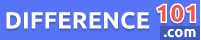Fluid Ounces

Fluid ounces (fl oz) is a unit of measurement for liquid volume commonly used in the United States and other countries that follow the US Customary system. It is defined as the volume of one fluid ounce of water under standard temperature and pressure conditions. One fluid ounce is equal to approximately 29.5735 milliliters (ml) or 0.125 cups. This unit of measure is commonly used for small quantities of liquids such as in recipes for cooking or baking, measuring medicine dosages, and measuring small amounts of liquids in scientific experiments. Understanding fluid ounces and its conversion ratios is important for accurate measurement and ensuring precise quantities of liquids are used in various applications